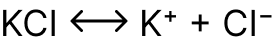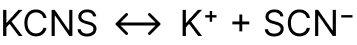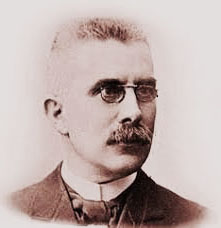Study the Shift in Equilibrium between Ferric ions and Thiocyanate ions

# Our Objective

To understand the shift in equilibrium between Ferric ions and Thiocyanate ions by either increasing/decreasing the concentration of the ions.

# The Theory

An unexpectedly disrupted equilibrium system will react oppositely until the balance is restored.

Let us study the equilibrium reaction between Potassium Thiocyanate and Ferric Chloride through the change in the concentration of colour of the solution. The reaction and equilibrium constant are given by

## Fe3+(aq) + SCN-(aq) ⇌ [Fe (SCN)]2+ (aq)

The formula gives the equilibrium constant.

## Kc = [C][D]/[A][B]

Finding the equilibrium constant for the reaction between Potassium Thiocyanate and Ferric Chloride

## K [[Fe (SCN)]2+(aq)]/[Fe3+(aq)[SCN−(aq)]] orAt a constant temperature, the value of K also remains constant.

Increasing the concentration of either Fe3+ ion or Thiocyanate ion would increase the concentration of [Fe (SCN)]2+ ions.

• If we add Ferric Chloride solution to the deep, red-coloured solution containing Ferric ions, Thiocyanate ions and Ferric thiocyanate complex, the concentration of Ferric ions increases. As a result, more Thiocyanate ions combine with Ferric ions to increase the [Fe (SCN)2+] complex; therefore, the red solution's colour intensity increases. The increase in the concentration of ferric ions shifts the equilibrium in the forward direction.
• If we increase Thiocyanate concentration, more Ferric ions react with Thiocyanate ions to increase the [Fe (SCN)2+] complex. As a result, the colour intensity of the red solution increases. Thus, the increase in the concentration of Thiocyanate ions shifts the equilibrium in the forward direction.
• If we add Potassium Chloride to the solution, the concentration of Potassium ions increases.It affects the equilibrium between Potassium ions and Thiocyanate ions.As the concentration of Potassium ions increases, more Thiocyanate ions react with Potassium ions to form more Potassium Thiocyanate and the above equilibrium shift in the backward direction occurs. As a result, the concentration of Thiocyanate ions decreases, and equilibrium shifts in the backwards direction. In other words, some of the [Fe(SCN)2+] complex dissociates to give Fe3+ and SCN-  ions.

As a result, the concentration of [Fe (SCN)2+] decreases and therefore, the intensity of the red colour decreases. Thus, the increase in the concentration of K+ ions shift the equilibrium in the backward direction.

## Law of Equilibrium?

The Law of Chemical Equilibrium is defined as the ratio of the product of the concentration of the products to the product of the concentration of the reactants, with each concentration term raised to the power by its coefficient in an overall balanced chemical equation; it is a constant quantity at a given temperature, and it is called equilibrium constant.

## What is Le Chatelier's principle?Le Chatelier, a French chemist in 1884, proposed that the effect of change in temperature, pressure and concentration on a system in equilibrium can be studied. Le Chatelier’s principle states that the new mixture is also called equilibrium when we add chemicals together than the number of molecules in each chemical. In a chemical reaction, there are always different numbers of atoms and molecules. There are some rules about how these numbers should change when you mix chemicals. For example, if two gases combine, the total gas amount must stay the same. That means the number of molecules in the mixture stays the same. This is known as chemical equilibrium.

Temperature, pressure, and concentration all affect the state of equilibrium in a system. Change to any of them, or change how much of each there is, and you’ll see equilibrium being disrupted. Equilibrium is restored when everything goes back to normal.

The principle of Le Chatelier is an observation regarding the chemical equilibria of processes. It asserts that changes in a system’s temperature, pressure, volume, or concentration will cause predictable and opposing changes to attain a new equilibrium state. In practice, Le Chatelier’s approach may be utilised to understand reaction circumstances that encourage enhanced product production.

## 2SO2(g)+O2(g)⇄2SO3(g); ΔH=−189kJ

Effect of Temperature: The above equilibrium reaction is exothermic because the enthalpy of production of SO3 is negative. When the temperature of the reaction is raised, the reaction will proceed in the direction of heat absorption, i.e., backwards. When the temperature is reduced, the equilibrium shifts forward, where the heat is released. As a result of its exothermic nature, low temperature promotes this reaction. In the Contact method, an ideal temperature of 673-723 K is employed for the production of SO3.

Pressure's effect: The given reaction proceeds with a decrease in the number of moles. If the pressure increases, the equilibrium, according to Le Chatelier, shifts in the direction where the pressure decreases. Thus, the number of moles decreases, i.e., in the forward direction. As a result, increasing pressure promotes the formation of SO3.

Effect of Concentration: As the concentrations of the reactants SO2 and O2 increase, the reaction equilibrium shifts in the direction where the reactants are consumed, i.e., forward, according to Le Chatelier's principle. As a result, an increase in SO2 and O2 concentration leads to an increase in SO3.

## Point to note

The reactions (in the gaseous phase) in which the number of moles of the product equals the number of moles of reactants, where equilibrium is pressure independent. Adding a catalyst does not affect the equilibrium position of a reaction.

# Learning Outcomes

• The experiment helps students understand the term "chemical equilibrium".
• Students comprehend the impact of concentration changes on the equilibrium of a reaction.
• Students comprehend the shift in equilibrium position due to concentration change.
• After experiencing it in the virtual lab, students can learn better experiments in the actual lab.